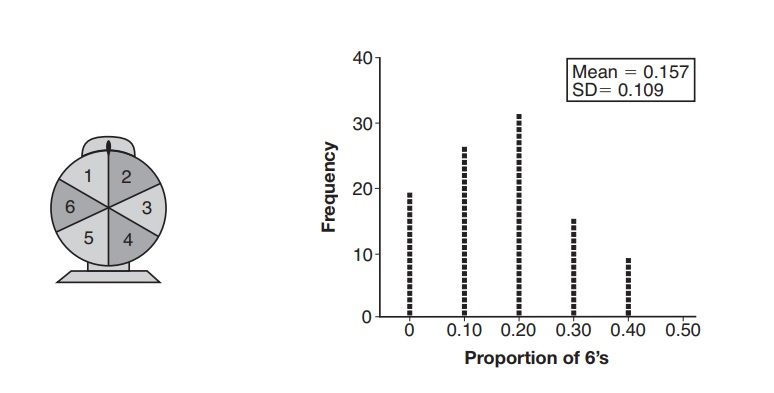## Wednesday, June 06, 2018

### Algebra 2 Problems of the Day

Daily Algebra 2 questions and answers.

More Algebra 2 problems.

June 2017, Part I

All Questions in Part I are worth 2 credits. No work need be shown. No partial credit.

10. A game spinner is divided into 6 equally sized regions, as shown in computations. the diagram below.
For Miles to win, the spinner must land on the number 6. After spinning the spinner 10 times, and losing all 10 times, Miles complained that the spinner is unfair. At home, his dad ran 100 simulations of spinning the spinner 10 times, assuming the probability of winning each spin is 1/6. The output of the simulation is shown in the diagram below.Which explanation is appropriate for Miles and his dad to make?
1) The spinner was likely unfair, since the number 6 failed to occur in about 20% of the simulations.
2) The spinner was likely unfair, since the spinner should have landed on the number 6 by the sixth spin.
3) The spinner was likely not unfair, since the number 6 failed to occur in about 20% of the simulations.
4) The spinner was likely not unfair, since in the output the player wins once or twice in the majority of the simulations.

Answer: 3) The spinner was likely not unfair, since the number 6 failed to occur in about 20% of the simulations.
Since the number 6 didn't show up in 20% of the simulations, it is not unreasonable that it wouldn't occur in 10 spins of a spinner, even if it seems to be unlikely.

11. Which binomial is a factor of x4 - 4x2 - 4x + 8?
1) x - 2
2) x + 2
3) x - 4
4) x + 4

Answer: 1) x - 2
If you graph the function, it has two zeroes, at (approximately) x = 1.13 and 2. So (x - 2) is a factor.

Alternatively, you could calculate to see if there would be a remainder. Luckily, the first choice is the answer.

12. Given that sin2θ + cos2θ = 1 and sin θ = - SQRT(2)/5, what is a possible value of cos θ?

Answer: 2) -0.15x3 - 0.02x2 + 28x - 120
If sin θ = -SQRT(2)/5, sin2θ = (-sqrt(2)/5)2 = 2/25.
This means that cos2θ = 23/25, and cos θ = SQRT(23/25), which is +Sqrt(23)/5.

Comments and questions welcome.

More Algebra 2 problems.